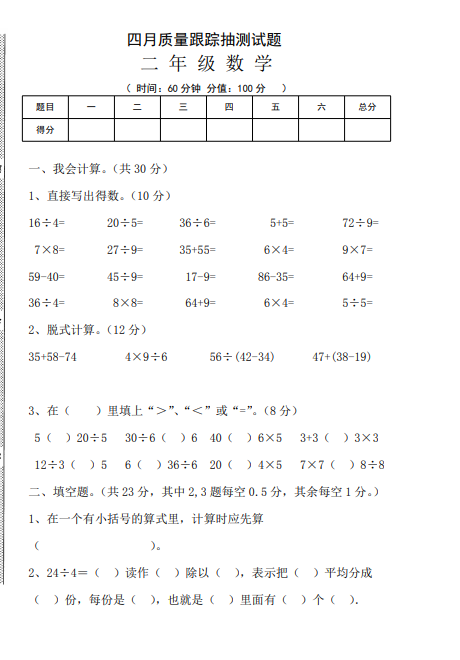（ 时间：60 分钟 分值：100 分 ）

1、直接写出得数。（10 分）
16÷4= 20÷5= 36÷6= 5+5= 72÷9=
7×8= 27÷9= 35+55= 6×4= 9×7=
59-40= 45÷9= 17-9= 86-35= 64+9=
36÷4= 8×8= 64+9= 6×4= 5÷5=

【PDF文档4页】人教版小学二年级数学下册第二次月考试题 (4月份)A4电子版资料_可直接打印_会员免费下载##### 评论信息